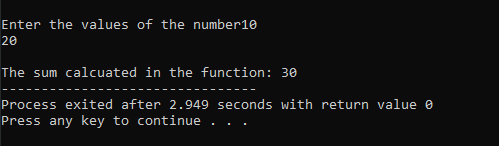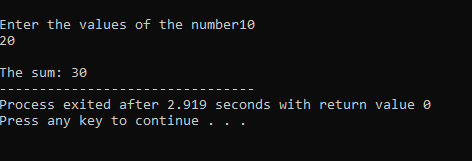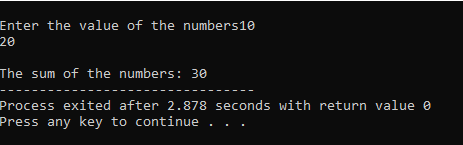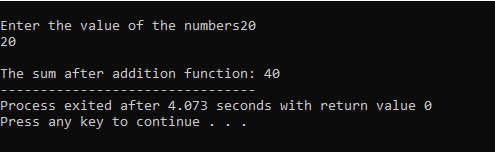# User-Defined functions in C

These are the types of functions that are designed by the user itself for certain specific purposes, we have already discussed functions in the earlier article.

There are 4 types of user defined functions, let us discuss them one by one.

## No argument and no return value:

In this type of function, no argument is passed in the program and there is no return value received at the end of the function.

For instance:

```#include<stdio.h>
int main()
{
return 0;
}
{
int a,b;
printf("\nEnter the values of the number");
scanf("%d%d",&a,&b);
int s=a+b;
printf("\nThe sum calcuated in the function: %d",s);
}```

In the above program, the add() is a user-defined function that has no return type and no arguments are passed in the function, hence shows the example of the above type of user-defined function.

Output:## No argument with a return type:

In this type of function, no argument is passed but the function has a return type i.e., a return value will be received at the end of the function.

For instance:

```#include<stdio.h>
int main()
{
printf("\nThe sum: %d",s);
return 0;
}
{
int a,b;
printf("\nEnter the values of the number");
scanf("%d%d",&a,&b);
int sum=a+b;
return sum;
}```

In the above program no argument is passed but the addition of the two number is stored in sum which is returned to the main function at the end of the user defined function, hence illustrating the above type of user defined function.

Output:## No return type with the argument:

In this type of function, there is no return value received at the end of the function but arguments are passed.

For instance:

```#include<stdio.h>
int main()
{
int a,b;
printf("\nEnter the value of the numbers");
scanf("%d%d",&a,&b);
return 0;
}
{
int sum=a+b;
printf("\nThe sum of the numbers: %d",sum);
}```

In the above program, the values of the variables a and b are passed as arguments in the function add to find the sum and there is no return value received. Hence illustrating the above type of user defined function.

Output:## With argument with return type:

In this type of user defined function, arguments are also passed and a return value is also received.

For instance:

```#include<stdio.h>
int main()
{
int a,b;
printf("\nEnter the value of the numbers");
scanf("%d%d",&a,&b);
printf("\nThe sum after addition function: %d",s);
return 0;
}
{
int sum=a+b;
return sum;
}```

In the above program, the values of the variables a and b are passed as arguments to the add function and their addition value is stored in sum which is returned to the main function in variables s which again is printed to display the value of the addition.

Output:Hence, we come to the end of the topic on user-defined function in C and its types. Hope we have cleared all your doubts.

If you are interested to publish articles please, WhatsApp us.##### Soham Malakar

Hello my name is Soham Malakar am currently pursuing BCA

Coding is one of my favorite hobbies.
I love to code and an enthusiast to learn different types of programming languages.
I love to get intoxicated in the world of technology and video games.
A quick learner possessing better results.

Articles: 40